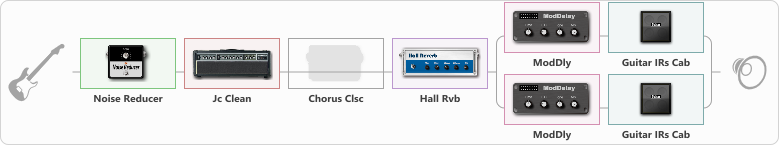# Stereo delay

Discussion in 'ToneLib-GFX presets' started by Ilya Masloboev, Apr 28, 2021.

Tags:
1. Stereo delay

Preset name: AMI Clean stereo delay

Effects chain:Effect: "Noise Reducer" (Dynamics / Filter), active - "yes"
{
"Sens" = 60
"Mode" = Soft
}

Effect: "Jc Clean" (Amp simulators), active - "yes"
{
"Gain" = 50
"Bass" = 80
"Middle" = 61
"Treble" = 48
"Presence" = 45
"Master" = 70
"Level (dB)" = 5
}

Effect: "Chorus Clsc" (Modulation / Sfx), active - "no"
{
"Speed" = 4.7
"Depth" = 58
"Center" = 1.0
"Mode" = Mono
}

Effect: "Hall Rvb" (Reverberation), active - "yes"
{
"Time" = 5.4
"PreDelay" = 0
"LoDamp" = 0
"HiDamp" = 17
"Mix" = 44
}

Effect: "Splitter" (Dynamics / Filter)
{
"A-Bypass" = Off
"A-Pan" = -57
"A-Level" = 55
"B-Bypass" = Off
"B-Pan" = 57
"B-Level" = 58

'A' branch:
{

Effect: "ModDly" (Delay), active - "yes"
{
"Time" = Note sync, 1/8 Dotted
"Feedback" = 58
"Tone" = 70
"Speed" = 1.6
"Mix" = 70
}

Effect: "Guitar IRs Cab" (Cabinets), active - "yes"
{
"Model" = Fender Deluxe Rev (1x12")
"Mic Position" = Middle
"Mic Distance" = Near
"Low Cut (Hz)" = 60
"Hi Cut (kHz)" = 20.0
"Mix" = 100
"Level (dB)" = 0
}
}
'B' branch:
{

Effect: "ModDly" (Delay), active - "yes"
{
"Time" = Note sync, 1/16 Dotted
"Feedback" = 68
"Tone" = 70
"Speed" = 1.6
"Mix" = 70
}

Effect: "Guitar IRs Cab" (Cabinets), active - "yes"
{
"Model" = Fender Twin Rev (2x12")
"Mic Position" = Middle
"Mic Distance" = Near
"Low Cut (Hz)" = 60
"Hi Cut (kHz)" = 20.0
"Mix" = 100
"Level (dB)" = 0
}
}
}

Note: You will need to download and install the ToneLib-GFX software to use the preset.

#### Attached Files:

• ###### Stereo_delay.tlgfx
File size:
892 bytes
Views:
2,626
2. If you have any wishes, do not hesitate to write